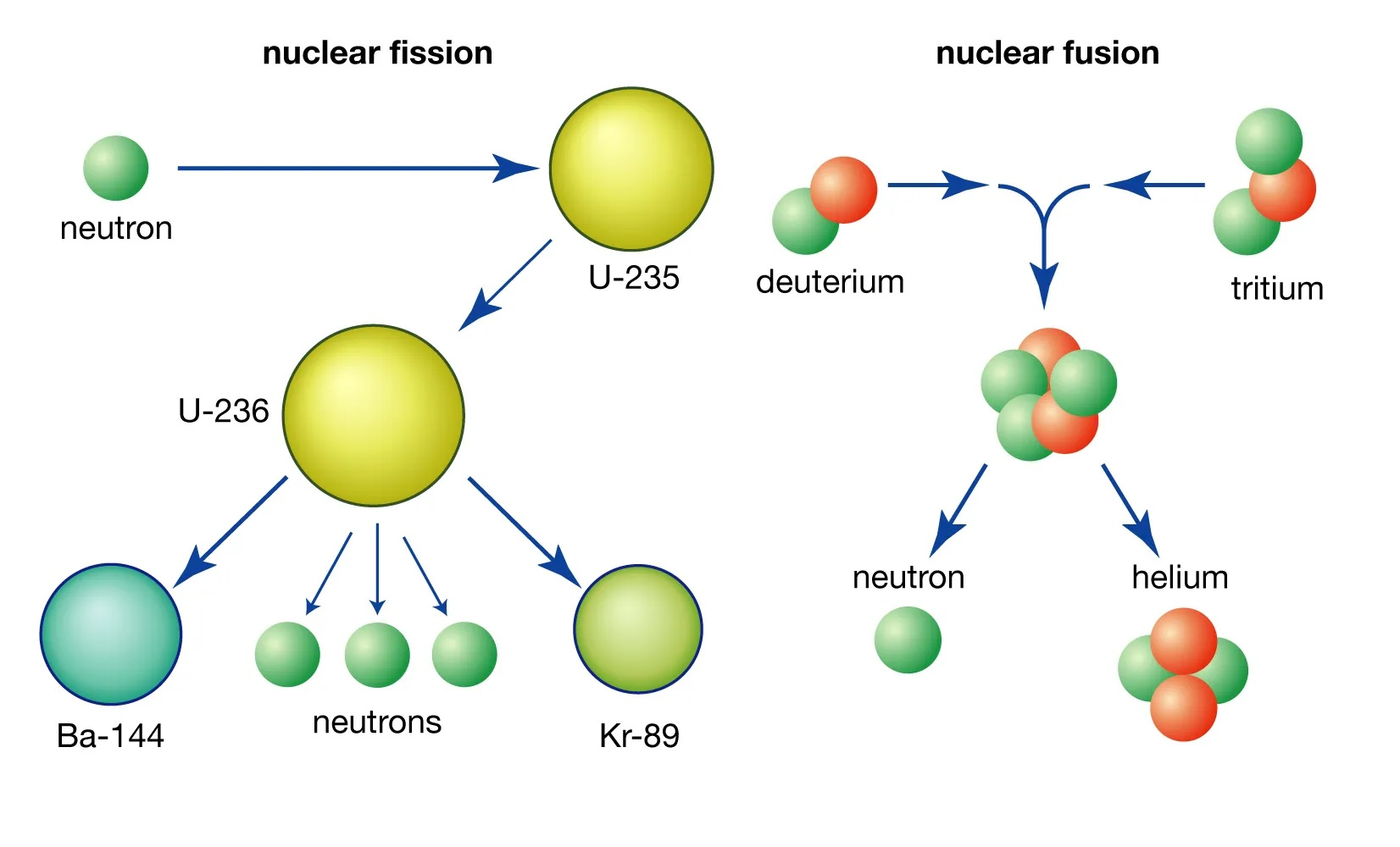## Latest Facts

### 9 Astounding Facts About CyclingOrelle Lenz

Published: 17 Sep 2023Source: Britannica.com

The concept of nuclear binding energy is a fascinating and crucial aspect of nuclear physics. It refers to the energy required to hold the nucleus of an atom together. Nuclear binding energy is responsible for the stability and characteristics of different elements on the periodic table. Understanding this concept is vital in various fields, including energy production, nuclear weapons, and even medicine.

In this article, we will explore 14 extraordinary facts about nuclear binding energy that will broaden your understanding of this intriguing subject. From the process of nuclear fusion to the famous equation E=mc² and its relation to binding energy, we will delve into the diverse and captivating aspects of this fundamental concept.

## The Binding Energy Per Nucleon is Maximum at Iron

The binding energy per nucleon reaches its maximum value at iron, making it the most stable element in terms of nuclear energy.

## Nuclear Fusion Releases Binding Energy

In nuclear fusion, when lighter atomic nuclei combine to form a heavier nucleus, the excess mass is converted into binding energy according to Einstein’s famous equation E=mc².

## Nuclear Fission is the Splitting of Atomic Nuclei

In nuclear fission, heavy atomic nuclei, such as uranium-235, are split into smaller fragments, releasing a significant amount of binding energy.

## Nuclear Power Plants Utilize Nuclear Fission

Nuclear power plants generate electricity by harnessing the energy released from nuclear fission reactions occurring in their reactors.

## The Mass Defect Determines Binding Energy

The mass defect, which is the difference between the combined mass of individual nucleons and the actual mass of the nucleus, determines the amount of binding energy released or required.

## Binding Energy Stabilizes Atomic Nuclei

The strong force, also known as the nuclear force, is responsible for binding protons and neutrons together in the nucleus, keeping the atom stable.

## Nuclear Binding Energy Enables the Sun’s Energy Production

In the core of the sun, fusion reactions occur, converting hydrogen into helium and releasing a tremendous amount of binding energy in the process.

## Isotopes Have Different Binding Energies

Different isotopes of the same element have slightly different binding energies due to variations in the number of neutrons in the nucleus.

## Binding Energy Can Determine Stability

Atoms with high binding energies tend to be more stable, while those with lower binding energies are prone to undergo radioactive decay.

## Binding Energy Allows for Nuclear Medicine

Nuclear medicine utilizes the emission of gamma rays resulting from the decay of radioactive isotopes, which is possible due to the release of binding energy.

## Mass Defect Can Lead to Nuclear Explosions

In nuclear weapons, the release of large amounts of binding energy during fission or fusion reactions leads to destructive explosions.

## Nuclear Binding Energy and the Periodic Table

The concept of binding energy helps explain the stability and arrangement of elements on the periodic table.

## Binding Energy Powers Nuclear Reactors

The sustained release and control of binding energy in nuclear reactors provide a reliable source of electricity for various industries.

## Nuclear Binding Energy and Nuclear Waste

Handling and disposing of nuclear waste is a significant challenge due to the high binding energy and long half-lives of radioactive isotopes.

These 14 extraordinary facts about nuclear binding energy highlight the importance of this concept in understanding atomic stability, nuclear reactions, and energy production. From nuclear power plants to the core of the sun, binding energy plays a crucial role in shaping our world.

## Conclusion

In conclusion, nuclear binding energy is a fascinating concept that plays a crucial role in the field of nuclear chemistry. Understanding the exceptional facts surrounding nuclear binding energy can provide valuable insights into the stability and energy release in atomic nuclei. The discovery and exploration of these extraordinary facts have revolutionized the way we harness nuclear energy for various applications, including power generation and medical therapies.

From the astonishingly high energy densities to the intricate relationship between mass and energy, nuclear binding energy continues to captivate scientists and researchers. The study of nuclear binding energy not only deepens our understanding of the fundamental forces that govern the universe but also holds the potential for groundbreaking discoveries and advancements in the future.

## FAQs

Q: What is nuclear binding energy?

A: Nuclear binding energy is the energy required to hold the nucleus of an atom together. It is the result of the strong nuclear force that overcomes the electromagnetic repulsion between the positively charged protons in the nucleus.

Q: How is nuclear binding energy calculated?

A: Nuclear binding energy is calculated by subtracting the combined masses of the individual nucleons (protons and neutrons) of an atom from the actual measured mass of the atom. The difference is then multiplied by the speed of light squared (E=mc^2) to convert the mass difference into energy.

Q: What are the main factors that influence nuclear binding energy?

A: The main factors that influence nuclear binding energy are the number of protons and neutrons in the nucleus, known as the mass number, and the type of nucleons present. Generally, nuclei with intermediate mass numbers have the highest binding energies.

Q: How is nuclear binding energy related to nuclear reactions?

A: Nuclear binding energy is released or absorbed during nuclear reactions. When lighter nuclei combine to form a heavier nucleus (fusion), the excess binding energy is released as a tremendous amount of energy. On the other hand, when a heavier nucleus splits into lighter fragments (fission), the released energy is also a result of the change in nuclear binding energy.

Q: What are some practical applications of nuclear binding energy?

A: The practical applications of nuclear binding energy are manifold. It is the basis for nuclear power generation, where the energy released during nuclear reactions is converted into electricity. Nuclear binding energy is also crucial in nuclear medicine, such as radiotherapy, where targeted radiation is employed to treat various diseases.